Trial ends in

A subscription to JoVE is required to view this content.

Rotational Inertia
##### Science Education (Physics I)
Hooke's Law and Simple Harmonic Motion
Entropy

# Rotational Inertia

### Overview

Source: Nicholas Timmons, Asantha Cooray, PhD, Department of Physics & Astronomy, School of Physical Sciences, University of California, Irvine, CA

Inertia is the resistance of an object to being accelerated. In linear kinematics, this concept is directly related to the mass of an object. The more massive an object, the more force is required to accelerate that object. This is seen directly in Newton's second law, which states that force is equal to mass times acceleration.

For rotation, there is a similar concept called rotational inertia. In this case, rotational inertia is the resistance of an object to being rotationally accelerated. Rotational inertia is dependent not only upon mass, but also upon the distance of mass from the center of rotation.

The goal of this experiment is to measure the rotational inertia of two rotating masses and to determine the dependence upon mass and distance from the axis of rotation.

### Principles

A certain object or system of objects has some rotational inertia. The rotational inertia about a certain axis is called the moment of inertia. Because the distance from the mass to the axis of rotation is important, a single object can have very different moments of inertia depending upon the axis about which it rotates. The moment of inertia for an object is defined as: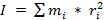, (Equation 1)

where i is the number of objects.

In Equation 1, r is the distance from the axis of rotation to the mass. As can be seen in the equation, the moment of inertia is dependent upon the mass of the object and the square of the distance from the mass to the axis of rotation.

Just like how linear kinematics has equations of motion, rotational kinematics has analogous equations of motion. For example, Newton's second law for linear motion is: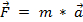. (Equation 2)

A similar rotational equation takes the form:, (Equation 3)

where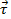is the torque,is the moment of inertia, and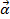is the angular acceleration. Here, the moment of inertia is the analog of the mass term in Newton's second law. Similarly, the moment of inertia is present in the other important equations of rotational motion: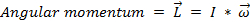, (Equation 4)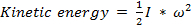, (Equation 5)

where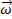is the angular velocity of the object.

For this experiment, a mass is connected to a rotating arm by a string wound around the axis of rotation. See Figure 1 for an image of what the experimental setup looks like. Two masses will be connected to the rotating arm, friction will be ignored in this experiment, and the total moment of inertia will be equal to the moment of the rotating masses plus the moment of the spinning arm.

The mass, which falls due to the influence of gravity, will enact a torque on the rotating arm. From Equation 2,and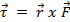. Here,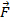is the force on the object, which comes from the tensionin the string, and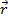is the distance from the force to the axis of rotation. Here, that distance is the distance from the edge of the wound string to the axis of rotation.

The angular accelerationis defined by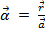, whereis the linear acceleration of a point on the wound string that corresponds to the acceleration of the falling weight. Putting everything together gives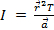. Newton's second law is used to find the tension. The sum of the forces on the object should be equal to the mass times the acceleration. Here, the forces on the falling weight are gravity (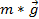) and the tension, so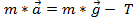. Assuming a constant acceleration, then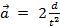, where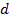is the distance the weight travels andis the time it takes to fall that distance. This comes from the kinematic equations of motion.

Putting everything together results in an equation for the moment of inertia in terms of quantities that are measurable during the experiment:. (Equation 7)

If two masses are attached to the spinning arm at equal distancesfrom the axis of rotation, then the moment of inertia will be: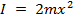, (Equation 8)

which is the theoretical value for this experiment.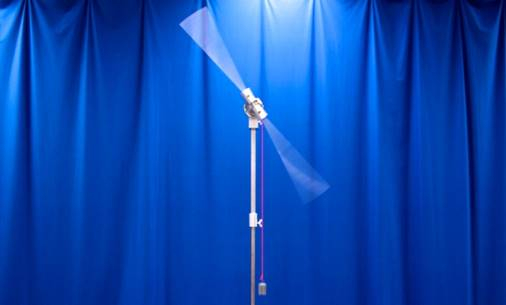Figure 1. Experimental setup.

### Procedure

1. Measure the moment of inertia of the long rod.

1. Wind the string attached to the weight until the weight is near the spinning arm.
2. Drop the weight and measure the time it takes to drop, as well as the distance it drops.
3. Perform step 1.2 three times and calculate the average moment of inertia using Equation 7.
4. Compute the theoretical moment of inertia of the spinning rod using the following formula: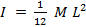, where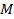is the mass of the rod andis the length.
5. Compare the theoretical value with the measured value and record the difference.

2. Two masses attached to the rod.

1. Place two 100-kg masses 20 cm away from the center of the rod.
2. Repeat steps 1.2 and 1.3 with the attached masses.
3. The total moment of inertia should be equal to the moment of inertia of the attached masses plus the moment of inertia of the rod. Use this fact, the results from step 1, and Equation 8 to determine the theoretical and experimental moments of inertia for the attached masses.
4. Compare the theoretical values with the measured values and record the differences.

3. Effect of distance on moment of inertia.

1. Repeat step 2 of the lab, but move the attached masses to 10 cm away from the center of rotation. Note any changes in the falling of the weight or the spinning of the rod.
2. Compare the theoretical values with the measured values and record the differences.

4. Effect of mass on the moment of inertia.

1. Repeat step 2 of the lab, but change the mass size to 200 kg.
2. Compare the theoretical values with the measured values and record the differences.

Rotational inertia characterizes the relationship between torque and an object's rotational acceleration.

Inertia is the resistance an object has to a change in its state of motion. In linear kinematics, the concept of inertia is directly related to the mass of an object. The more massive an object, the more force is required to accelerate that object.

In rotational kinematics, the concept is termed as rotational inertia, which is the resistance of an object to being rotationally accelerated. Rotational inertia, denoted by letter I, is dependent not only on the mass but also on the distance of the mass from the center of rotation, or r. And mathematically, it is given by the formula I equals m*r-square.

Note that if there is more than one rotating object, then the rotational inertia of the whole system is the sum of the individual rotational inertias -- given by this formula where lowercase i is for number of objects undergoing rotation.

This video will show how to theoretically and experimentally measure the rotational inertia of a spinning arm with and without attached masses.

Before going into the details of the protocol, let's talk about the experimental set-up and the laws and equations that govern rotational inertia in this system.

The first set-up consists of an axle, which is free to rotate around an axis of rotation. Then there is a weight attached to a string and the string is wound around the axle, such that the weight is close to the rod.

When the weight is released, the tension in the string provides the force for the rod to spin. The rotational inertia, also known as moment of inertia or angular mass or I of this rod can be experimental calculated using this formula. Here, r is the radius of the axle, m is the mass of the falling object, t is the time the object requires to fall to a measured distance d, and g is the acceleration due to gravity

Theoretically, the moment of inertia of any cylindrical rod is given by this formula, where M is the mass of the rod and L is the length of the rod.

In the next experiment, we will wind the string back, and attach two identical masses to the rod at the same distance x from the center. These two masses have their own moment of inertia, theoretically given by the formula I equals two times m x-square.

Now when the weight is released, the rod will spin again. In this case, the experimental inertia of the system-given by the previously discussed formula- will take into account both, the inertia of the two masses and the inertia of the rod. Therefore, subtracting the rod's inertia obtained in the first experiment from this value, will yield the experimental rotational inertia of just the masses in this system.

Now that you understand how to theoretically and experimental calculate the rotational inertias for the elements of this system, let's see how to set-up the experiment and how to record the values

As discussed, the first experiment measures the moment of inertia of the spinning rod alone. Take the string that is attached to the weight and wind it around the axle until the weight is close to the arm. Drop the weight. Measure and record the distance it falls and the time it takes to fall.

Wind the string and drop the weight three more times. Use the results from these trials to calculate the average moment of inertia for the spinning rod, then calculate the theoretical value.

The next set of experiment requires placing additional masses on the rod. Place two 1-kilogram masses on opposite sides of the rod, with each 20 centimeters from the center.

Wind the string around the axle until the weight is close to the arm. As before, release the weight and measure the distance it falls and the time it takes to fall. Repeat this procedure three more times.

With these experimental results, calculate the average total moment of inertia for the spinning rod with attached masses.

To study the effect of distance on the moment of inertia, reposition the 1 kilogram masses so they are each 10 centimeters from the center of the rod.

Perform the experimental procedure four times and notice any effect on the spin rate. Calculate the new average moment of inertia for only the masses and record the result.

Lastly, to analyze the effect of mass on the moment of inertia, change the two masses so they are each 2 kilograms and reposition them so they are 20 centimeters from the center of the rod.

Perform the experimental procedure four times and again notice any change in the behavior of the spinning rod. Calculate the new average moment of inertia for only the masses and record the result.

Theoretical and experimental values for the moment of inertia of the rod, and of the attached masses alone, agree reasonably well, confirming the equations describing rotational inertia. Limitations in measurement accuracy explain the percentage difference between expected and actual results.

Because moment of inertia is proportional to mass, the result for the 1 kilogram masses positioned 20 centimeters from the axis of rotation is half that of the 2 kilogram masses at the same distance.

Moment of inertia for the spinning masses is also proportional to the square of distance from the axis of rotation. The 1 kilogram masses located 20 centimeters from the center have twice the distance and, as expected, four times the moment of inertia compared to the same masses at 10 centimeters.

Rotational inertia is an important effect and it can be used advantageously in many situations.

A tightrope walker carries a long pole to increase his moment of inertia compared to using only his arms. Because of greater rotational inertia, the pole remains steady and horizontal, allowing the tightrope walker to stay balanced

The wheels of a car or any vehicle concentrate most of their mass on the outer side while keeping the center relatively lightweight. This hoop-like configuration is not only lighter but also has less rotational inertia than a solid disk.

As a result, less torque is needed to spin and stop the wheel, reducing demands on the engine when accelerating, as well as decelerating.

You've just watched JoVE's introduction to rotational inertia. You should now understand what moment of inertia is and how it depends on mass and distance from the center of rotation. As always, thanks for watching!

### Results

 Theoretical Value (kg m2) Experimental Value (kg m2) Difference (%) Part 1 0.20 0.22 10 Part 2 0.08 0.07 14 Part 3 0.02 0.02 0 Part 4 0.16 0.15 6

The results from the experiment confirm the predictions made by Equations 7 and 8. The moment of inertia for a spinning rod, as given by the formula in step 1.4, was experimentally confirmed. The reduced distance in step 3 resulted in a smaller moment of inertia, as predicted. The larger mass in step 4 resulted in a larger moment of inertia, as predicted by Equation 8.

### Applications and Summary

Have you ever wondered why a tightrope walker carries a very long pole? The reason is that the long pole has a very large moment of inertia due to its length. Therefore, it requires a large amount of torque to make it rotate. This helps the tightrope walker to stay balanced, as the pole will remain steady.

Wheels of cars and bicycles are never just solid disks; instead, they have spokes that support the wheel from the axle. This allows for a lighter design, which aids with speed, However, the real reason for this design can be explained rotational inertia. A solid disk has a larger moment of inertia than a hoop-like shape. With its smaller moment of inertia, a hoop requires less torque to spin and, perhaps more importantly, requires less torque to stop spinning.

When a baseball player is at bat against a pitcher throwing fastballs, he may want to speed up his swing in order to get a hit. He can achieve this by simply moving his hands closer to the heavy end of the bat, which is called "choking up." This reduces the distance from the center of mass of the bat to the axis of rotation and therefore makes it easier for the batter to rotate the bat.

In this experiment, the moment of inertia for a rod and two masses were experimentally measured and theoretically calculated. The differences between these values were examined. The effect of mass on the moment of inertia was tested, as well as the effect of distance from the axis of rotation.X